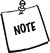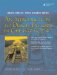The keyword operator is used in C++ to define a new meaning for an operator symbol such as +, -, =, *, &, and so forth. Adding a new meaning to an operator symbol is a specialized form of function overloading.

Operator overloading provides a more compact syntax for calling functions, which can lead to more readable code (assuming the operators are used in ways that are commonly understood).

It is possible to overload nearly all of the existing operator symbols in C++. For example, suppose that we want to define a class named Complex to represent complex numbers. To specify how to do the basic arithmetic operations with these objects we could overload the four arithmetic operator symbols. While we are at it, we could also overload the insertion symbol so that output statements become more readable.

 Complex numbers were introduced initially to describe the solutions to equations such as x2 6x + 25 = 0. Using the quadratic formula one can easily determine that the roots of this equation are 3 + 4i and 3 4i. The complex numbers consist of all numbers of the form a + bi, where a and b are real numbers and i is the square root of 1. Since that set includes such numbers for which b = 0, it is clear that the real numbers are a subset of the complex numbers.

Example 5.6 shows a class definition with both members and non-member operators.

Example 5.6. src/complex/complex.h

 ```#include using namespace std; class Complex { // binary non-member friend function declarations friend ostream& operator<<(ostream& out, const Complex& c); friend Complex operator-(const Complex& c1, const Complex & c2); friend Complex operator*(const Complex& c1, const Complex & c2); friend Complex operator/(const Complex& c1, const Complex & c2); public: Complex(double re = 0.0, double im = 0.0); // binary member function operators Complex& operator+= (const Complex& c); Complex& operator-= (const Complex& c); Complex operator+(const Complex & c2); <-- 1 private: double m_Re, m_Im; }; ```   (1)This should be a non-member friend like the other non-mutating operators.

The operators declared in Example 5.6 are all binary (accept 2 operands). For the member functions, there is only one formal parameter because the first (left) operand is implicit: *this. The member operators definitions are shown in Example 5.7.

Example 5.7. src/complex/complex.cpp

 ```[ . . . . ] Complex& Complex::operator+=(const Complex& c) { m_Re += c.m_Re; m_Im += c.m_Im; return *this; } Complex Complex::operator+(const Complex& c2) { return Complex(m_Re + c2.m_Re, m_Im + c2.m_Im); } Complex& Complex::operator-=(const Complex& c) { m_Re -= c.m_Re; m_Im -= c.m_Im; return *this; } ```

Example 5.8 shows the definitions of the non-member friend functions. They are defined like ordinary global functions.

Example 5.8. src/complex/complex.cpp

 ```[ . . . . ] ostream& operator<<(ostream& out, const Complex& c) { out << '(' << c.m_Re << ',' << c.m_Im << ')' ; return out; } Complex operator-(const Complex& c1, const Complex& c2) { return Complex(c1.m_Re - c2.m_Re, c1.m_Im - c2.m_Im); } ```

We have expressed the mathematical rules that define each of the four algebraic operations in C++ code. These details are encapsulated and hidden so that client code does not need to deal with them. Example 5.9 shows some client code that demonstrates and tests the Complex class.

Example 5.9. src/complex/complex-test.cpp

 ```#include "complex.h" #include int main() { using namespace std; Complex c1(3.4, 5.6); Complex c2(7.8, 1.2); cout << c1 << " + " << c2 << " = " << c1 + c2 << endl; cout << c1 << " - " << c2 << " = " << c1 - c2 << endl; Complex c3 = c1 * c2; cout << c1 << " * " << c2 << " = " << c3 << endl; cout << c3 << " / " << c2 << " = " << c3 / c2 << endl; cout << c3 << " / " << c1 << " = " << c3 / c1 << endl; return 0; } ```

Here is the output of the program in Example 5.9:

```(3.4,5.6) + (7.8,1.2) = (11.2,6.8)
(3.4,5.6) - (7.8,1.2) = (-4.4,4.4)
(3.4,5.6) * (7.8,1.2) = (19.8,47.76)
(19.8,47.76) / (7.8,1.2) = (3.4,5.6)
(19.8,47.76) / (3.4,5.6) = (7.8,1.2)
```

## Member versus Global Operators

As we have seen, it is possible to overload operators as member functions, or as global functions. The primary difference that you will notice first is how they can be called. In particular, a member function operator requires an object as the left operand. A global function, in contrast, permits the same kinds of type conversions for either operand.

Example 5.10 shows why Complex::operator+() would be better suited as a non-member function.

Example 5.10. src/complex/complex-conversions.cpp

 ```#include "complex.h" int main() { Complex c1 (4.5, 1.2); Complex c2 (3.6, 1.5); Complex c3 = c1 + c2; Complex c4 = c3 + 1.4; <-- 1 Complex c5 = 8.0 - c4; <-- 2 Complex c6 = 1.2 + c4; <-- 3 } ```   (1)Right operand is promoted. (2)Left operand is promoted. (3)Error: Left operand is not promoted for member operators.

There are some limitations on operator overloading. Only built-in operators can be overloaded. It is not possible to introduce definitions for symbols such as \$, ", or ' that do not already possess operator definitions. Although new meanings can be defined for built-in operators, their associativity and precedence remain the same.

It is possible to overload all of the built-in binary and unary operators except for these:

• The ternary conditional operator testExpr ? valueIfTrue : valueIfFalse
• Scope resolution operator ::
• Member select operators . and .*Here is one way to remember which operators can be overloaded: If it has a dot in it (.) anywhere, it's probably not allowed.Overloading the comma operator is allowed, but not recommended until you are a C++ expert.

We have provided a complete table of operator symbols and their characteristics in Section 19.1.

 1 Continue the development of the Fraction class by adding overloaded operators for addition, subtraction, multiplication, division, and various kinds of comparison. In each case the parameter should be a const Fraction&. Write client code to test your new operators. 2 To be really useful, a Fraction object should be able to interact with other kinds of numbers. Expand the definition of Fraction so that the operators in Exercise 1 also work for int and double. It should be clear, for example, how to get frac + num to be correctly evaluated. How would you handle the expression num + frac, where frac is a Fraction and num is an int? Write client code to test your new functions. 3 Add arithmetic and comparison operators to the class Complex. Write client code to test your expanded class.An Introduction to Design Patterns in C++ with Qt 4
ISBN: 0131879057
EAN: 2147483647
Year: 2004
Pages: 268

Similar book on Amazon